The first three blog posts in my “Deep Learning Paper Implementations” series will cover Spatial Transformer Networks introduced by Max Jaderberg, Karen Simonyan, Andrew Zisserman and Koray Kavukcuoglu of Google Deepmind in 2016. The Spatial Transformer Network is a learnable module aimed at increasing the spatial invariance of Convolutional Neural Networks in a computationally and parameter efficient manner.

In this first installment, we’ll be introducing two very important concepts that will prove crucial in understanding the inner workings of the Spatial Transformer layer. We’ll first start by examining a subset of image transformation techniques that fall under the umbrella of affine transformations, and then dive into a procedure that commonly follows these transformations: bilinear interpolation.

In the second installment, we’ll be going over the Spatial Transformer Layer in detail and summarizing the paper, and then in the third and final part, we’ll be coding it from scratch in Tensorflow and applying it to the GTSRB dataset (German Traffic Sign Recognition Benchmark).

For the full code that appears on this page, visit my Github Repository.

### Image Transformations

To lay the groundwork for affine transformations, we first need to talk about linear transformations. To that end, we’ll be restricting ourselves to 2 dimensions and work with matrices.

We define the following:

• a point K with coordinates $\begin{bmatrix} x \\ y \end{bmatrix}$ represented as a $(2\times1)$ column vector.
• a matrix $% $ represented as a square matrix of shape $(2\times2)$.

and would like to examine the linear transformation $T$ defined by the matrix product $K' = T(K) = MK$ as we vary the parameters a, b, c and d of M.

Warm-Up Question.

Say we set $a = d = 1$ and $b = c = 0$ as follows:

In that case, what transform do you think we would obtain? Go ahead and give it a few moment’s thought…

Solution.

Let’s write it out:

We’ve actually represented the identity transform, meaning that the point K does not move in the plane. Let us now jump to more interesting transforms.

Scaling.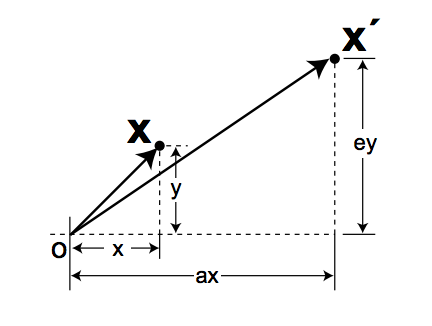We let $b = c = 0$, and $a$ and $d$ take on any positive value.

Note that there is a special case of scaling called isotropic scaling in which the scaling factor for both the x and y direction is the same, say $s$. In that case, enlarging an image would correspond to $s > 1$ while shrinking would correspond to $% $. It’s a bit non-intuitive then that to zoom-in on an image, you need $% $ (think about it).

Anyway, performing the matrix product, we obtain

Rotation.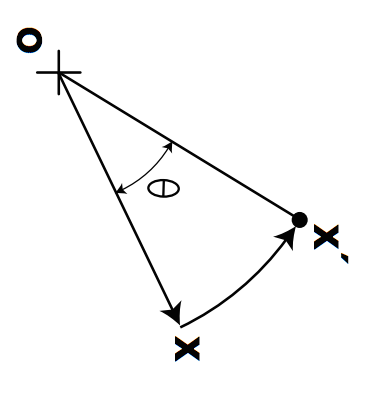Suppose we want to rotate by an angle $\theta$ about the origin. To do so, we set $a = d = \cos{\theta}$ and $b = c = \sin{\theta}$ as follows:

We thus obtain

Shear.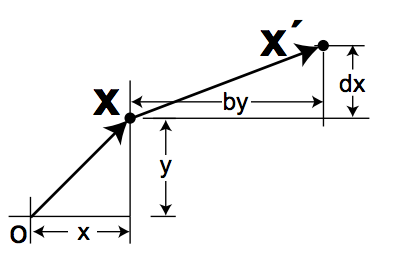When we shear an image, we offset the y direction by a distance proportional to x, and the x direction by a distance proportional to y. For example, when we go from normal text to italics, we are effectively applying a shear transform (think about shearing a deck of cards if that helps).

To achieve shearing, we set $a = d = 1$, $b = m$ and $c = n$ as follows:

This yields

In summary, we have defined 3 basic linear transformations:

• scaling: scales the x and y direction by a scalar.
• shearing: offsets the x by a number proportional to y and x by a number proportional to x.
• rotating: rotates the points around the origin by an angle $\theta$.

Now the nice thing about matrices is that we can collapse sequential linear transformations into a single transformation matrix. For example, say we would like to apply a shear, a scale and then a rotation to our column vector K. Given that these transformations can be represented by the matrices $H$, $S$ and $R$, and respecting the order of transformations, we can write down this operation as

But recall that matrix multiplication is associative! So this reduces to

where $M = RSH$. Be mindful of the order since matrix multiplication $\color{red}{\text{is not}}$ commutative.

A beautiful consequence of this formula is that if we are given multiple transformations to do for a very high-dimensional vector, then we can basically carry out a single matrix multiplication rather than repeatedly manipulating the high-dimensional vector for every sequential transformation.

Translation.

The only downside to this $2 \times 2$ matrix representation is that we cannot represent translation since it isn’t a linear transformation. Translation however, is a very important and needed transformation, so we would like to be able to encapsulate it in our matrix representation.

To solve this dilemna, we represent our 2D vectors in 3D using homogeneous coordinates as follows:

• our point K becomes a $(3\times1)$ column vector $\begin{bmatrix} x \\ y \\ 1 \end{bmatrix}$
• our matrix M becomes a $(3\times3)$ square matrix $% $

To represent a translation, all we have to do is place 2 new parameters $e$ and $f$ in our third column like so

and we can thus carry out translations as linear transformations in homogeneous coordinates. Note that if we require a 2D output, then all we need to do is represent M as a $2 \times 3$ matrix and leave K untouched.

Example.

Translate both the x and y direction by $\Delta$. Result should be 2D.

Summary.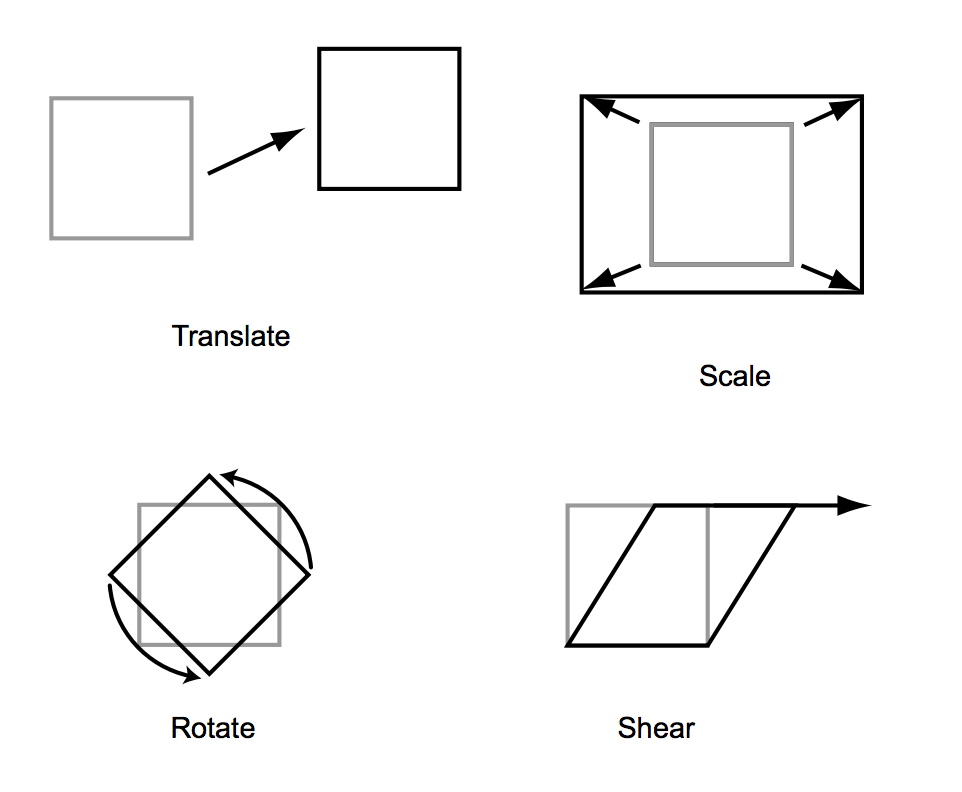By using a little trick, we were able to add a new transformation to our repertoire of linear transformations. This transformation, called translation, is an affine transformation. Hence, we can generalize our results and represent our 4 affine transformations (all linear transformations are affine) by the 6 parameter matrix

### Bilinear Interpolation

Motivation. When an image undergoes an affine transformation such as a rotation or scaling, the pixels in the image get moved around. This can be especially problematic when a pixel location in the output does not map directly to one in the input image.

In the illustration below, you can clearly see that the rotation places some points at locations that are not centered in the squares. This means that they would not have a corresponding pixel value in the original image.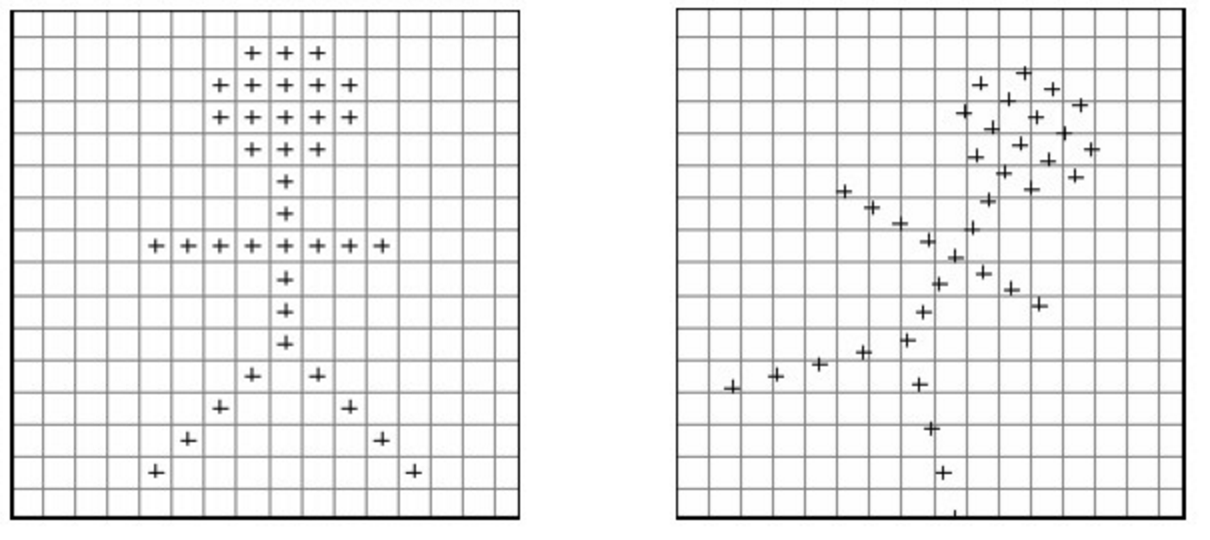So for example, suppose that after rotating an image, we need to find the pixel value at the location (6.7, 3.2). The problem with this is that there is no such thing as fractional pixel locations.

To solve this problem, bilinear interpolation uses the 4 nearest pixel values which are located in diagonal directions from a given location in order to find the appropriate color intensity values of that pixel. The result is smoother and more realistic images!

Algorithm.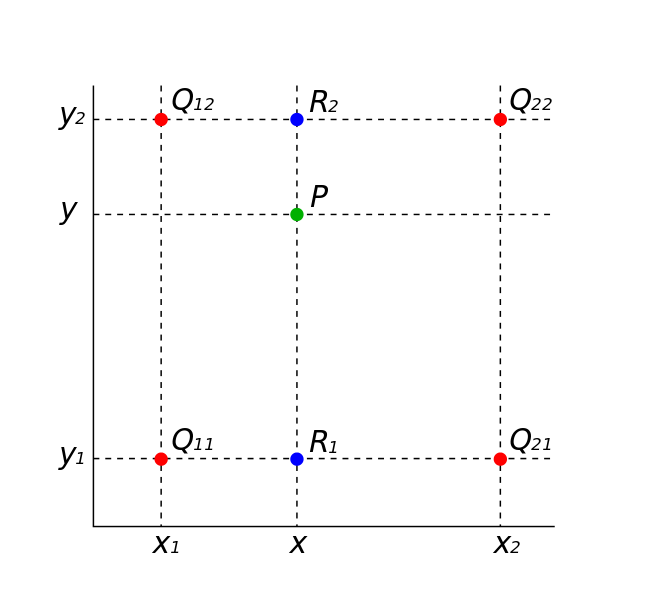Our goal is to find the pixel value of the point P. To do so, we calculate the pixel value of $R_1$ and $R_2$ using a weighted average of $(Q_{11}, Q_{21})$ and $(Q_{12}, Q_{22})$ respectively. Then, we use a weighted average of $R_2$ and $R_1$ to find the value of P.

Effectively, we are interpolating in the x direction and then the y direction, hence the name bilinear interpolation. You could just as well flip the order of interpolation and get the exact same value.

So given a point $P = (x, y)$ and 4 corner coordinates $Q_{11} = (x_1, y_1)$, $Q_{21} = (x_2, y_1)$, $Q_{12} = (x_1, y_2)$ and $Q_{22} = (x_2, y_2)$, we first interpolate in the x-direction:

and finally in the y-direction:

Python Code.

One very very important note before we jump into the code!

An image processing affine transformation usually follows the 3-step pipeline below:

• First, we create a sampling grid composed of $(x, y)$ coordinates. For example, given a 400x400 grayscale image, we create a meshgrid of same dimension, that is, evenly spaced $x \in [0, W]$ and $y \in [0, H]$.
• We then apply the transformation matrix to the sampling grid generated in the step above.
• Finally, we sample the resulting grid from the original image using the desired interpolation technique.

As you can see, this is different than directly applying a transform to the original image.

I’ve attached 2 cat images in the Github Repository mentioned at the top of this page which you should go ahead and download. Save them to your Desktop in a folder called data/ or make sure to update the path location if you choose differently.

I’ve also written a function load_img() that converts images to numpy arrays. I won’t go into its details but it’s pretty basic and you shouldn’t take long to understand what it does. Note that you’ll need both PIL and Numpy to reproduce the results below.

Armed with this function, let’s load both cat images and concatenate them into a single input array. We’re working with 2 images because we want to make our code as general as possible.

import numpy as np
from PIL import Image

# params
DIMS = (400, 400)
CAT1 = 'cat1.jpg'
CAT2 = 'cat2.jpg'

# concat into tensor of shape (2, 400, 400, 3)
input_img = np.concatenate([img1, img2], axis=0)

# dimension sanity check
print("Input Img Shape: {}".format(input_img.shape))


Given that we have 2 images, our batch size is equal to 2. This means that we need an equal amount of transformation matrices M for each image in the batch.

Let’s go ahead and initialize 2 identity transform matrices. This is the simplest case, and if we implement our bilinear sampler correctly, we should expect our output image to be almost exact to the input image.

# grab shape
num_batch, H, W, C = input_img.shape

# initialize M to identity transform
M = np.array([[1., 0., 0.], [0., 1., 0.]])

# repeat num_batch times
M = np.resize(M, (num_batch, 2, 3))


(Recall that our general affine transformation matrix is $2 \times 3$ if we want to include translation.)

Now we need to write a function that will generate a meshgrid for us and output a sampling grid resulting from the product of this meshgrid and our transformation matrix M.

Let’s go ahead and generate our meshgrid. We’ll create a normalized one, that is the values of x and y range from -1 to 1 and there are width and height of them respectively. In fact, note that for images, x corresponds to the width of the image (i.e. number of columns of the matrix) while y corresponds to the height of the image (i.e. number of rows of the matrix).

# create normalized 2D grid
x = np.linspace(-1, 1, W)
y = np.linspace(-1, 1, H)
x_t, y_t = np.meshgrid(x, y)


Then we need to augment the dimensions to create homogeneous coordinates.

# reshape to (xt, yt, 1)
ones = np.ones(np.prod(x_t.shape))
sampling_grid = np.vstack([x_t.flatten(), y_t.flatten(), ones])


So we’ve created 1 grid here, but we need num_batch grids. Same as above, our one-liner below repeats our array num_batch times.

# repeat grid num_batch times
sampling_grid = np.resize(sampling_grid, (num_batch, 3, H*W))


Now we perform step 2 of our image transformation pipeline.

# transform the sampling grid i.e. batch multiply
batch_grids = np.matmul(M, sampling_grid)
# batch grid has shape (num_batch, 2, H*W)

# reshape to (num_batch, height, width, 2)
batch_grids = batch_grids.reshape(num_batch, 2, H, W)
batch_grids = np.moveaxis(batch_grids, 1, -1)


Finally, let’s write our bilinear sampler. Given our coordinates x and y in the sampling grid, we want interpolate the pixel value in the original image.

Let’s start by seperating the x and y dimensions and rescaling them to belong in the height/width interval.

x_s = batch_grids[:, :, :, 0:1].squeeze()
y_s = batch_grids[:, :, :, 1:2].squeeze()

# rescale x and y to [0, W/H]
x = ((x_s + 1.) * W) * 0.5
y = ((y_s + 1.) * H) * 0.5


Now for each coordinate $(x_i, y_i)$ we want to grab 4 corner coordinates.

# grab 4 nearest corner points for each (x_i, y_i)
x0 = np.floor(x).astype(np.int64)
x1 = x0 + 1
y0 = np.floor(y).astype(np.int64)
y1 = y0 + 1


(Note that we could just as well use the ceiling function rather than the increment by 1).

Now we must make sure that no value goes beyond the image boundaries. For example, suppose we have $x = 399$, then $x_0 = 399$ and $x_1 = x0 + 1 = 400$ which would result in a numpy error. Thus we clip our corner coordinates in the following way:

# make sure it's inside img range [0, H] or [0, W]
x0 = np.clip(x0, 0, W-1)
x1 = np.clip(x1, 0, W-1)
y0 = np.clip(y0, 0, H-1)
y1 = np.clip(y1, 0, H-1)


Now we use advanced numpy indexing to grab the pixel value for each corner coordinate. These correspond to (x0, y0), (x0, y1), (x1, y0) and (x_1, y_1).

# look up pixel values at corner coords
Ia = input_img[np.arange(num_batch)[:,None,None], y0, x0]
Ib = input_img[np.arange(num_batch)[:,None,None], y1, x0]
Ic = input_img[np.arange(num_batch)[:,None,None], y0, x1]
Id = input_img[np.arange(num_batch)[:,None,None], y1, x1]


Almost there! Now, we calculate the weight coefficients,

# calculate deltas
wa = (x1-x) * (y1-y)
wb = (x1-x) * (y-y0)
wc = (x-x0) * (y1-y)
wd = (x-x0) * (y-y0)


and finally, multiply and add according to the formula mentioned previously.

# add dimension for addition
wa = np.expand_dims(wa, axis=3)
wb = np.expand_dims(wb, axis=3)
wc = np.expand_dims(wc, axis=3)
wd = np.expand_dims(wd, axis=3)

# compute output
out = wa*Ia + wb*Ib + wc*Ic + wd*Id


### Results

So now that we’ve gone through the whole code incrementally, let’s have some fun and experiment with different values of the transformation matrix M.

The first thing you need to do is copy and paste the whole code which has been made more modular. Now let’s test if our function works correctly.

Identity Transform.

Add the following 2 lines as the end of the script and execute.

plt.imshow(out)
plt.show()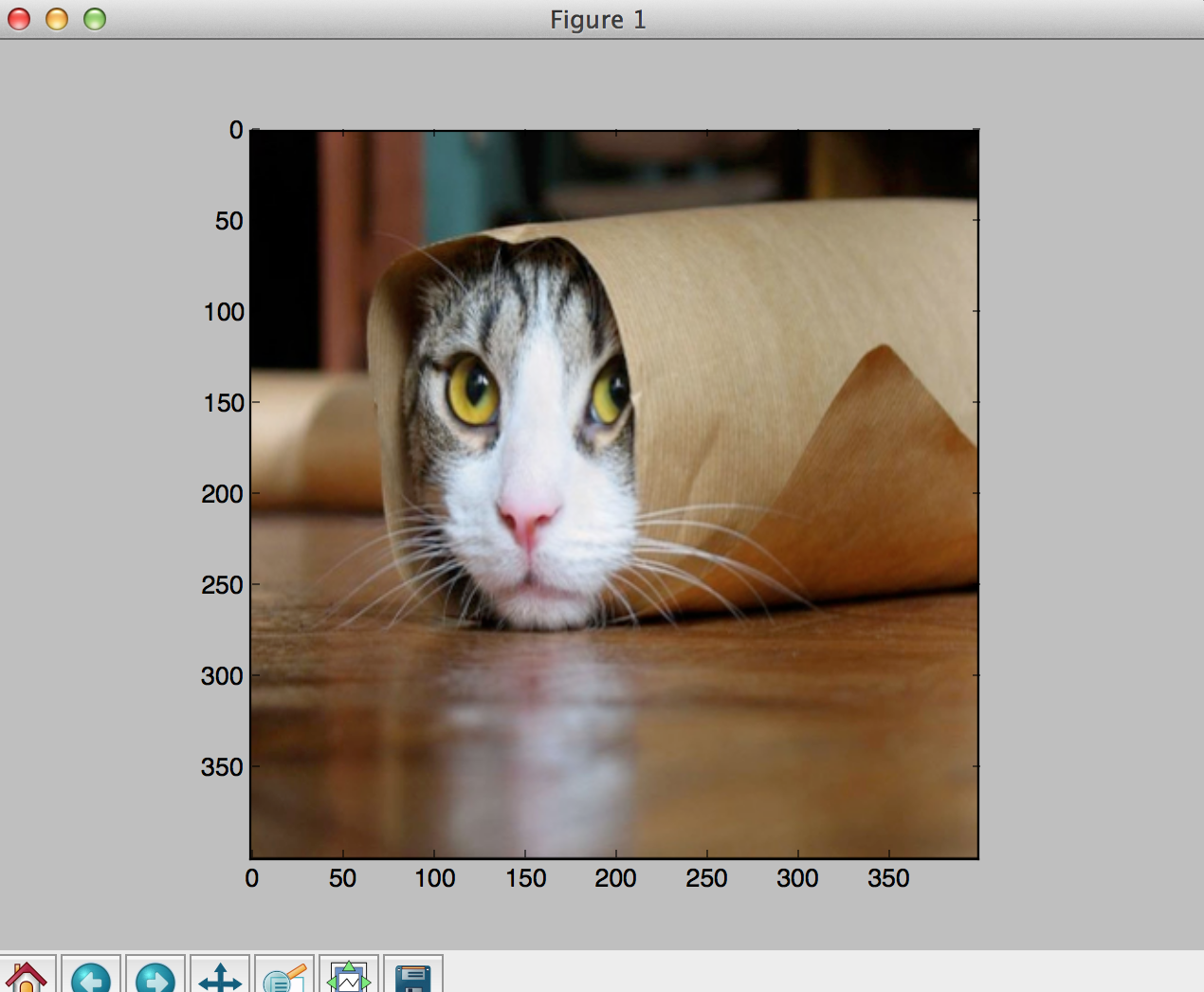Translation.

Say we want to translate the picture by 0.5 only in the x direction. This should shift the image to the left.

Edit the following line of your code as follows:

M = np.array([[1., 0., 0.5], [0., 1., 0.]])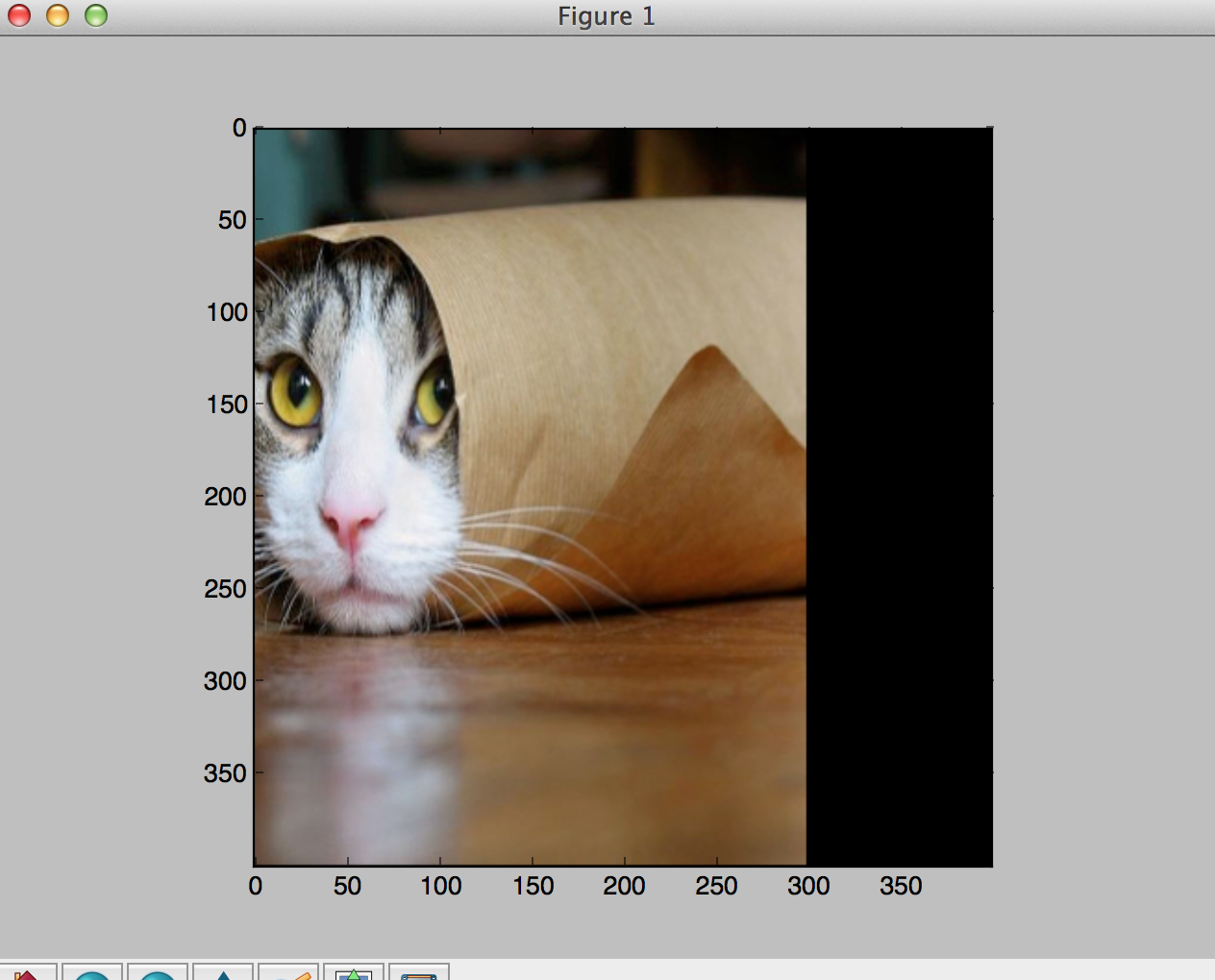Rotation.

Finally, say we want to rotate the picture by 45 degrees. Given that $\cos{(45)} = \sin{(45)} = \frac{\sqrt{2}}{2} \approx 0.707$, edit just this line of your code as follows:

M = np.array([[0.707, -0.707, 0.], [0.707, 0.707, 0.]])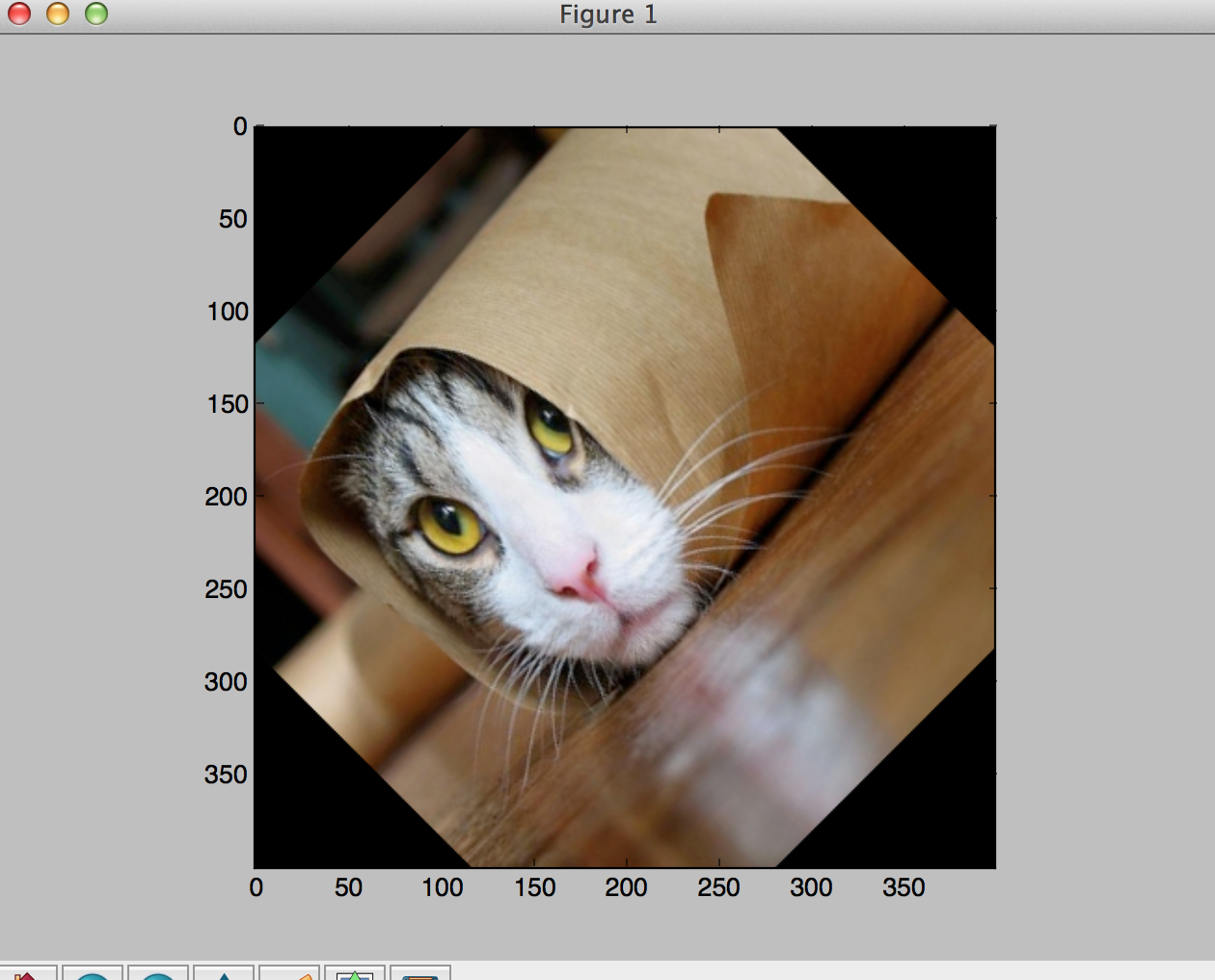### Conclusion

In this blog post, we went over basic linear transformations such as rotation, shear and scale before generalizing to affine transformations which included translations. Then, we saw the importance of bilinear interpolation in the context of these transformations. Finally, we went over the algorithm, coded it from scratch in Python and wrote 2 methods that helped us visualize these transformations according to a 3 step image processing pipeline.

In the next installment of this series, we’ll go over the Spatial Transformer Network layer in detail as well as summarize the paper it is described in.

See you next week!

A big thank you to Eder Santana for introducing me to this paper!